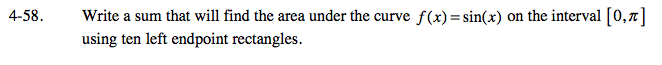### Home > PC > Chapter 4 > Lesson 4.1.4 > Problem4-58

4-58.$\frac{\text{interval length}}{\text{rectangles}} \ \ \ \frac{\pi}{10}$

$\frac{\pi}{10}\displaystyle\sum\limits_{k=0}^9$

$\text{sin}(\ \ \ \ \ \ )$

$\text{sin}\left(\frac{\pi}{10}\textit{k}\right)$